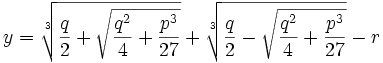## Sunday, January 06, 2008

### De Moivre: Fifth Root of Unity and the Seventh Root of Unity

Abraham de Moivre was able to solve Φ5 and Φ7 [see here for Definition of Cyclotomic Polynomial if needed] in order to express the primitive 5th roots of unity and the primitive 7th roots of unity as radicals.

In today's blog, I will show de Moivre's solutions.

Theorem 1: fifth root of unity can be solved by radicals.

Proof:

(1) Since 5 is prime, from a previous result (see Lemma 1, here), we have:

Φ5 = x4 + x3 + x2 + x + 1 = 0

(2) Dividing both sides by x2, the gives us:

x2 + x + 1 + x-1 + x-2 = 0

(3) Let y = x + x-1

(3) Then:

y2 = (x + x-1)2 = x2 + 2x*x-1 + x-2 = x2 + 2 + x-2

(4) So,

y2 + y - 1 = x2 + x-2 + x + x-1 + 1

(5) Using the quadratic equation to solve for y2 + y - 1 = 0 gives us (see Theorem, here if needed):

y = (-1 ± √1 + 4)/2 = (-1 ± √5)/2

(6) Since y = x + x-1, we have:

x2 - [(-1 ± √5)/2]x + 1 = 0

(7) Again, using the quadratic equation, we have:

x = { [(-1 ± √5)/2] ± √[(-1 ± √5)/2]2 - 4}/2

(8) We can simplify this by noting that:

[(-1 ± √5)/2]2 = [1 ± 2√5 + 5]/4 = [6 ± 2√5]/4

(9) So,

x = { [(-1 ± √5)/2] ± √[(-1 ± √5)/2]2 - 4}/2 =

= [(-1 ± √5)/2 ± √(6 ± 2√5)/4 - 16/4]/2 =

= [(-1 ± √5)/2 ± √(-10 ± 2√5)/4]/2 =

= [(-1 ± √5) ± √(-10 ± 2√5)]/4

QED

Theorem 2: Seventh root of unity can be solved by radicals.

Proof:

(1) Since 7 is prime, from a previous result (see Lemma 1, here), we have:

Φ7 = x6 + x5 + x4 + x3 + x2 + x + 1 = 0

(2) Dividing both sides by x3, the gives us:

x3 + x2 + x + 1 + x-1 + x-2 + x-3 = 0

(3) Let y = x + x-1

(3) Then:

y2 = (x + x-1)2 = x2 + 2xx-1 + x-2 = x2 + 2 + x-2

y3 = (x + x-1)(x2 + 2 + x-2) = x3 + 2x + x-1 + x + 2x-1 + x-3 = x3 + 3x + 3x-1 + x-3

(4) So,

y3 + y2 - 2y - 1 = x3 + 3x + 3x-1 + x-3 + x2 + 2 + x-2 -2(x + x-1) - 1 =

= x3 + x2 + (3x - 2x) + (2 -1) + (3x-1 - 2x-1) + x-2 + x-3 =

= x3 + x2 + x + 1 + x-1 + x-2 + x-3

(5) So using the cubic equation (see Theorem, here), we can solve for y in:

y3 + y2 - 2y - 1 = 0

to get:where

p = (-2)/(1) - (1)2/3(1)2 = -2 - (1/3) = -7/3

q = (-2(1)3)/(27(1)3) + (1)(-2)/(3(1)2) + 1/1 = -2/27 -2/3 + 1 = (-2 -18 + 27)/27 = 7/27

r = (1)/(3) = 1/3

(6) Once a solution for y is found, then it is possible to solve for x using the quadratic equation:

x2 - [(-1 ± √5)/2]y + 1 = 0

QED

It would have to wait until Alexandre-Theophile Vandermonde to solve for the eleventh root of unity in terms of radicals. Later, Carl Friedrich Gauss would demonstrate that all roots of unity are expressible as radicals.

References
• Jean-Pierre Tignol, Galois' Theory of Algebraic Equations, World Scientific, 2001